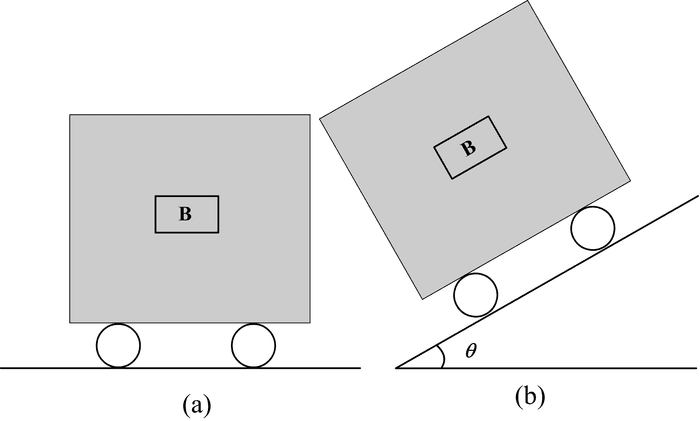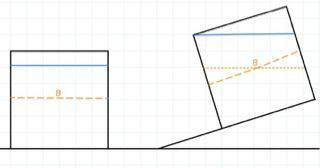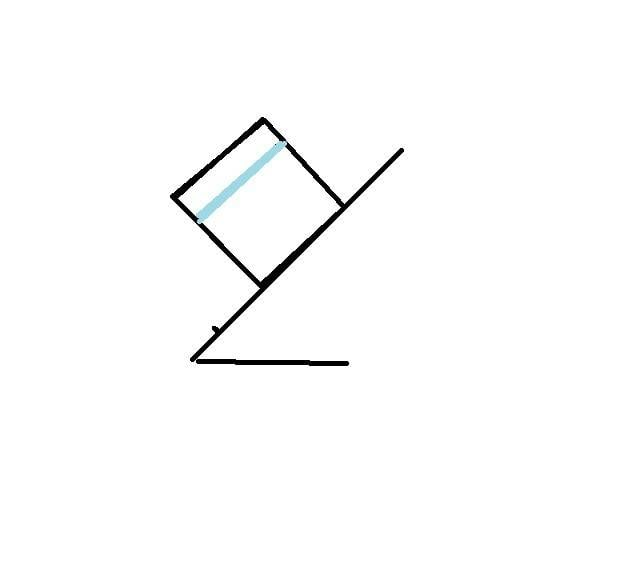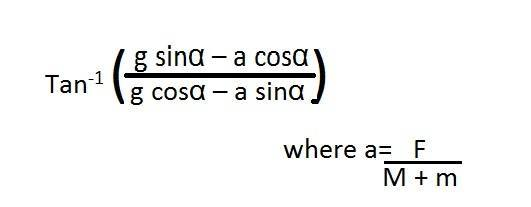# Fluids acceleration on inclined plane

Missing homework template due to originally being posted elsewhere.
A body is capable of remaining stationary inside a liquid in a closed box (that means its density is same as that of liquid) . If the whole system is placed on inclined plane and allowed to slide down then:
1. body moves up relative to liquid
2. moves down relative to liquid
3. stationary wrt to liquid
4. move up for some angle and move down for some other angle..

ATTEMPT
at first i thought density is same so body doesnot move relative to liquid but the answer is 4.... can anyone explain.....??Bystander
Homework Helper
Gold Member
its density is same as that of liquid
i.e., it is indistinguishable from the liquid for purposes of this experiment, and remains ...
3. stationary wrt to liquid
There may have been a previous version of the question in which the box was not filled completely, and the answer key has not been brought up to date.

but what will be the shape of free surface of liquid....
and suppose free surface of liquid comes below the box then i think box will have to move down...

in which the box was not filled completely
suppose it was partially filled then can you explain it please

Bystander
Homework Helper
Gold Member
The drawing/sketch of the problem shows liquid filling the box, so it can't be moved relative to the liquid.
suppose it was partially filled
On a frictionless plane, it still won't move. Every volume increment of water is accelerating at the same rate.
With friction, it still doesn't move.
This is beginning to look very much like an "intuitive solution" to a counter-intuitive analytical result.

•jonny23
thanks....but will there be any case when the block moves up or down ...if so plz tell

Bystander
Homework Helper
Gold Member
When the box is not fully filled, and someone starts rocking it back and forth setting up waves, the block will follow the wave motion, but other than that, unless we start postulating compressible fluids or a compressible block, nothing --- watch wait staff in restaurants carrying beverages sometime.

•jonny23
I think it will rotate and move down because the force that is holding the body up in the liquid is pressure and pressure is a function of the depth ,namely H, when the box is put on an inclined plane, to have the same pressure ,the depth must increase because (sign theta times H is smaller than H)

Nathanael
Homework Helper
I think it will rotate and move down because the force that is holding the body up in the liquid is pressure and pressure is a function of the depth ,namely H, when the box is put on an inclined plane, to have the same pressure ,the depth must increase because (sign theta times H is smaller than H)
Buoyancy doesn't depend on depth.

to have the same pressure ,the depth must increase

the buyount force doesnot depend on absolute pressure rather it depends on pressure difference and it will be same

haruspex
Homework Helper
Gold Member
the buyount force doesnot depend on absolute pressure rather it depends on pressure difference and it will be same
You're right that it doesn't depend on depth (ignoring any compressibility of the fluid), but thinking of it as depending on pressure difference is a bit awkward. Pressure difference between where and where, exactly? If you want to express it in terms of pressure, you would need to consider the integral of the pressure over the surface, bearing in mind that the surface area elements are vectors: ##\int_S P \vec{dA}##. More useful, probably, to stick with the usual 'weight of the fluid displaced' formulation.

Btw, if we consider compressibility, a body that manages to sit in the middle of a fluid under neutral buoyancy by virtue of having not just the same density as the fluid but also the exact same compressibility is physically unrealistic. More reasonably, the body is less compressible than the fluid, allowing it to sit stably at the depth where the densities match. This may admit a different answer to the question, though maybe none of those offered.

•jonny23
usual 'weight of the fluid displaced' formulation.

so for a non compressible ideal fluid can the body B rotate or move ??

haruspex
Homework Helper
Gold Member
so for a non compressible ideal fluid can the body B rotate or move ??
If neither the fluid nor the object are compressible, and they have the same density, there is no reason why the acceleration of the box down the slope would cause the object to move within the fluid. You can arrive at this by a simple thought experiment. Imagine the object as just a notional parcel of the fluid within a box full of fluid. Why should that parcel move relative to the rest of the fluid?
Of course, in tipping the box prior to letting it slide down the slope, the body might change its orientaion relative to the box.

•jonny23I misused the word 'depth'. well in comparison to the liquid surface the position of the body doesn't change but it does change in comparison to the top of the box.
In this illustration the blue line shows the liquid level, the Orange dotted line shows the position of the body and the dashed – line shows the position in comparison to the top of the box.

haruspex
Homework Helper
Gold Member
View attachment 77129

I misused the word 'depth'. well in comparison to the liquid surface the position of the body doesn't change but it does change in comparison to the top of the box.
In this illustration the blue line shows the liquid level, the Orange dotted line shows the position of the body and the dashed – line shows the position in comparison to the top of the box.
Why do you assume (a) that the fluid does not fill the box and (b) that the top of the liquid will be level as the box accelerates down the slope?

That is only for stationary box, actually I don't understand the question quite well!

That is only for stationary box

ya it will be horizontal when stationary but please explain what happens when it accelerates

haruspex
Homework Helper
Gold Member
That is only for stationary box,
Ok, but looking at distance from the top of the sloping box isn't relevant. The question asks how/if the object moves relative to the liquid.
please explain what happens when it accelerates
Perhaps the easiest way to see how it will behave is to consider a small blob of the liquid at its surface. It is surrounded by other liquid below and 360 degrees around. So which way does the net force on it from the rest of the liquid point?

i guess that it will be parallel to inclined surface .as the net force should be perpendicular to liquid free surface and the so liquid sholud be parallel to surface.am i right?

haruspex
Homework Helper
Gold Member
i guess that it will be parallel to inclined surface .as the net force should be perpendicular to liquid free surface and the so liquid sholud be parallel to surface.View attachment 77176 am i right?
In the absence of friction on the slope, yes - well done.
(Can you work out what would happen with some kinetic friction?)

it should be tan^-1(u) with the incline...

haruspex
Homework Helper
Gold Member
it should be tan^-1(u) with the incline...
How do you arrive at that? I get something a bit different.

Edit: I get the same now.Last edited:
i guess that it will be parallel to inclined surface .as the net force should be perpendicular to liquid free surface and the so liquid sholud be parallel to surface.View attachment 77176 am i right?
Why would this happen?

How do you arrive at that? I get something a bit different.

in frame of the vessel we apply pseduo force on a liquid particle that is ma = mgsin(thetha) - u mgcos(thetha) and the downward force i.e. mg .

The resultant of the two should be perpendicular to the surface.

haruspex
Homework Helper
Gold Member
Why would this happen?
For the reasons given. If the body of water is unchanging in shape as the box slides down the ramp, a parcel of water at the surface must have forces acting on it to result in the same acceleration. This implies a matching slope to the surface.

TSny
Homework Helper
Gold Member
it should be tan^-1(u) with the incline...
That looks correct to me.

An interesting modification of the problem is to allow the incline to slide on a frictionless, horizontal surface. Suppose a horizontal force of arbitrary magnitude is applied to the incline. If there is no friction between the box and the incline, what is the orientation of the surface of the water? (A special case of this would be to let F = 0 so that the incline recoils to the right as the box slides down the slope.) I get a simple answer (for arbitrary F, M, and m).

#### Attachmentsi got this

TSny
Homework Helper
Gold Member

That's what I get if the box does not slip along the incline (due to sufficient static friction, say). But if there is no friction between the box and the incline, then generally the box will either slide down or up the incline depending on the strength of the force F.

haruspex
Homework Helper
Gold Member
Up to about post #13, the thread was mostly in relation to the original problem. Nobody could come up with an explanation for the supposed correct answer. Instead, the consensus is that the body would not move relative ro the liquid.
After that, the thread broadened into interesting discussion of how the liquid would behave if it does not fill the box, under various conditions of friction and applied forces.

But if there is no friction between the box and the incline, then generally the box will either slide down or up the incline depending on the strength of the force F.
this is the general equation which i derived.
See following CASES :
if a=0 you see the equation gives angle of free surface as (alpha)
if a< g tan(alpha) where (alpha) is angle of inclined plane, then block moves downward wrt to inclined plane and free surface is given as per equation
if a =g tan(alpha) block is stationary wrt to the plane and the angle comes out to be zero which should be (as in post #14)
if a> g tan (alpha) block moves upward with respect to the plane and the angle comes out to be negative

TSny
Homework Helper
Gold Member
If a represents the acceleration of the incline, then I agree with your conclusions about which way the block will slide along the incline. However, I get a simpler answer for the orientation of the surface of the water. I think you can get the answer with very little calculation. Go to the non-inertial reference frame of the block and consider a free body diagram of the block. In this frame there is an effective acceleration of gravity, ##\small \vec{g}_{eff}##, that can be expressed in terms of the true acceleration of gravity ##\small \vec{g}## and the acceleration of the block relative to the earth, ##\small \vec{a}_{block}##. From the free body diagram of the block, can you determine the direction of ##\small \vec{g}_{eff}## without first working out an expression for ##\small \vec{a}_{block}##?

Last edited:
then i am getting tan^-1 (a/g)
But i think its not right because if you put a =0 then the free surface would be horizontal but shouldnt it be paralllel to incline?

TSny
Homework Helper
Gold Member
then i am getting tan^-1 (a/g)

But i think its not right because if you put a =0 then the free surface would be horizontal but shouldnt it be paralllel to incline?

Right, we know that the answer for a = 0 should be that the surface is parallel to the incline. Not sure how you got tan^-1 (a/g).

256bits
Gold Member
Up to about post #13, the thread was mostly in relation to the original problem. Nobody could come up with an explanation for the supposed correct answer. Instead, the consensus is that the body would not move relative ro the liquid.
After that, the thread broadened into interesting discussion of how the liquid would behave if it does not fill the box, under various conditions of friction and applied forces.
Problem states that the closed box is allowed to slide down, not that it is sliding down the ramp. I interpret this to mean it eventually ends up moving horizontally.

At the curve the box will experience a dw/dt, a change in dy/dt and a change in dx/dt.
It could be that with different angles of incline, the differences in these values will cause the object to shift its position within the box.